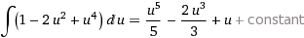Posted on

# Calculus Test 4 Sections 4.9-5.8 – #3, #8b, #9a, #9b, #10b questions

Question from Beverly:

#3 –  on the test copy I have says to use three equal subintervals.  But in the handwritten solution, it appears that they used 6. If the student uses 3 subintervals wouldn’t the ∆X be 2 and the right endpoint sum be 0.2, left end point be 39.8, and midpoint be 15.8?

Answer from Dr Callahan – That is correct – in the solution we used an interval of 1 and it should have been 2.

#8b – The handwritten solution has dV= √t and V = t^1.5.  Shouldn’t V = 2/3*t^1.5? With that, our final solution came out to be 3.39.

Answer from Dr Callahan – That is also correct – we have an error in our solution.

#9a – Instead of factoring the cos^5x into (cos^2x)(cos^2x)cosx, my student used the Form 74 from the Table of Integrals followed by Form 68. But he didn’t get the same answer as he would have solving it as we learned in 5.7 (p. 403) like the written solution did. Why don’t the two methods yield the same answer? I’m thinking it has to do with the limits of the integral, but it’s been 25 years since I had calculus, and I’m struggling with explaining the why on this one.

Answer from Dr Callahan – Yes you should get the same answer – but I noticed an error in our solution!!!! When you integrate we added a 4. Should be as below#9b – In the handwritten solution it looks like it evaluates 3*ln 3 as 9.89, but isn’t it 3.3? I believe the answer would be -0.17 if 3.3 is correct.

Answer from Dr Callahan –  Yes it should be 3.3

Extra Credit – The solution just substituted into the Form #95.  Did you not expect them to continue to solve the remaining integral or just stop with that first substitution?

Answer from Dr Callahan – Yes we just stopped there because that was the challenging part!